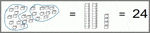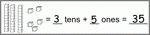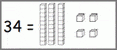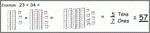# 1st Grade Base 10 Blocks Worksheets

## Grade 1 base ten blocks worksheets

Our first grade base ten blocks worksheets help students understand the base 10 number system by composing and de-composing numbers into their base 10 constituents. These worksheets only use "tens" and "ones" (no hundreds of thousands).

## Regrouping unit blocks into blocks of 10In these base 10 block regrouping worksheets, the student is given a number of blocks, regroups then into tens and ones and writes the total as a two-digit number.  This exercise gives practice in counting and emphasizes the relationship between the groups of blocks and the corresponding digits in a two-digit number.

## Counting using base 10 blocksOur base 10 blocks counting worksheets show the student a number of blocks and ask him or her to determine the total number of blocks by counting "tens" and "ones".  No regrouping is required. Only tens and ones are used (no hundreds or thousands).

## Breaking a number into tens and onesWe have 5 decomposing numbers into base 10 blocks worksheets, where the student is given a two digit number and represents it pictorially by drawing rods (tens) and blocks (ones). Numbers up to 99 are used.

## Addition with base 10 blocks5 addition with base 10 blocks worksheets: students are asked to add two two-digit numbers together using base 10 blocks.  The student draws blocks to represent each number, then re-draws the blocks added together.  From the new diagram, the student can determine the sum. This exercise lays the foundation for column addition of two digit numbers.  The final two worksheets involve some regrouping of ones into tens.

## More place value & rounding worksheets

Explore all of our  place value worksheets, from base ten blocks to 6 digit numbers in expanded form, and our  rounding worksheets, ranging from rounding to the nearest 10 to rounding in the millions.

## What is K5?

K5 Learning offers reading and math worksheets, workbooks and an online reading and math program for kids in kindergarten to grade 5.  We help your children build good study habits and excel in school.